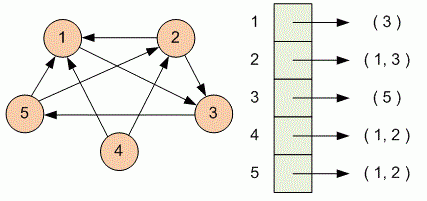Problems

A simple directed graph is given with an adjacency matrix. Print its representation in the form of adjacency list.

#### Input

First line contains the number of vertices in a graph n (1n100). Then the adjacency matrix is given. It is guaranteed that graph does not contain loops.

#### Output

Print n lines - the adjacency list of the graph. Print in the i-th line the number of edges adjacent to the i-th vertex, and then the vertex numbers where these edges go in increasing order.Time limit 1 second
Memory limit 128 MiB
Input example #1
5
0 0 1 0 0
1 0 1 0 0
0 0 0 0 1
1 1 0 0 0
1 1 0 0 0

Output example #1
1 3
2 1 3
1 5
2 1 2
2 1 2